## Probability distribution.

The interval $$\left[0,1\right]$$ is a subset of $$\\R$$. B is a continuous random variables with probability $$p\left( 0\le B\le 1 \right) =\quad 1\\$$ in one dimension.

Considere a point (B,C) in a plane where the density of probability is:

$$\\f\left[ \left( B,C \right) \right] =\begin{cases} 1\quad if\quad (B,C)\quad \epsilon \quad \left[ 0,1 \right] { \times \left[ 0,1 \right] } \\ 0\quad otherwise \end{cases}\\$$

The density function is well defined, then B and C form a proper probability distribution.

Instead of B and C I will use x and y.$$\\p\left[ \left( 0\le x\le 1 \right) and\left( 0\le y\le 1 \right) \right] \quad =\quad 1$$ or We have $${ \int { f(x,y) } }_{ { \left[ 0,1 \right] }^{ 2 } }\quad =\quad 1\\$$

Find the probability that:

1. x + y < 1/2 That is y = -x + 1/2 We are interested of the area under the line.
x <- c(0:1000/1000)
y <- -1*x + 1/2
a <- c(0:1000/2000)
b <- ifelse(a+y<2,t<-a+y,s<-a+y)
qplot(x, y, xlim = c(0,1),ylim = c(0,1)) + geom_area(fill = "Yellow")
## Warning: Removed 500 rows containing missing values (position_stack).
## Warning: Removed 500 rows containing missing values (geom_point).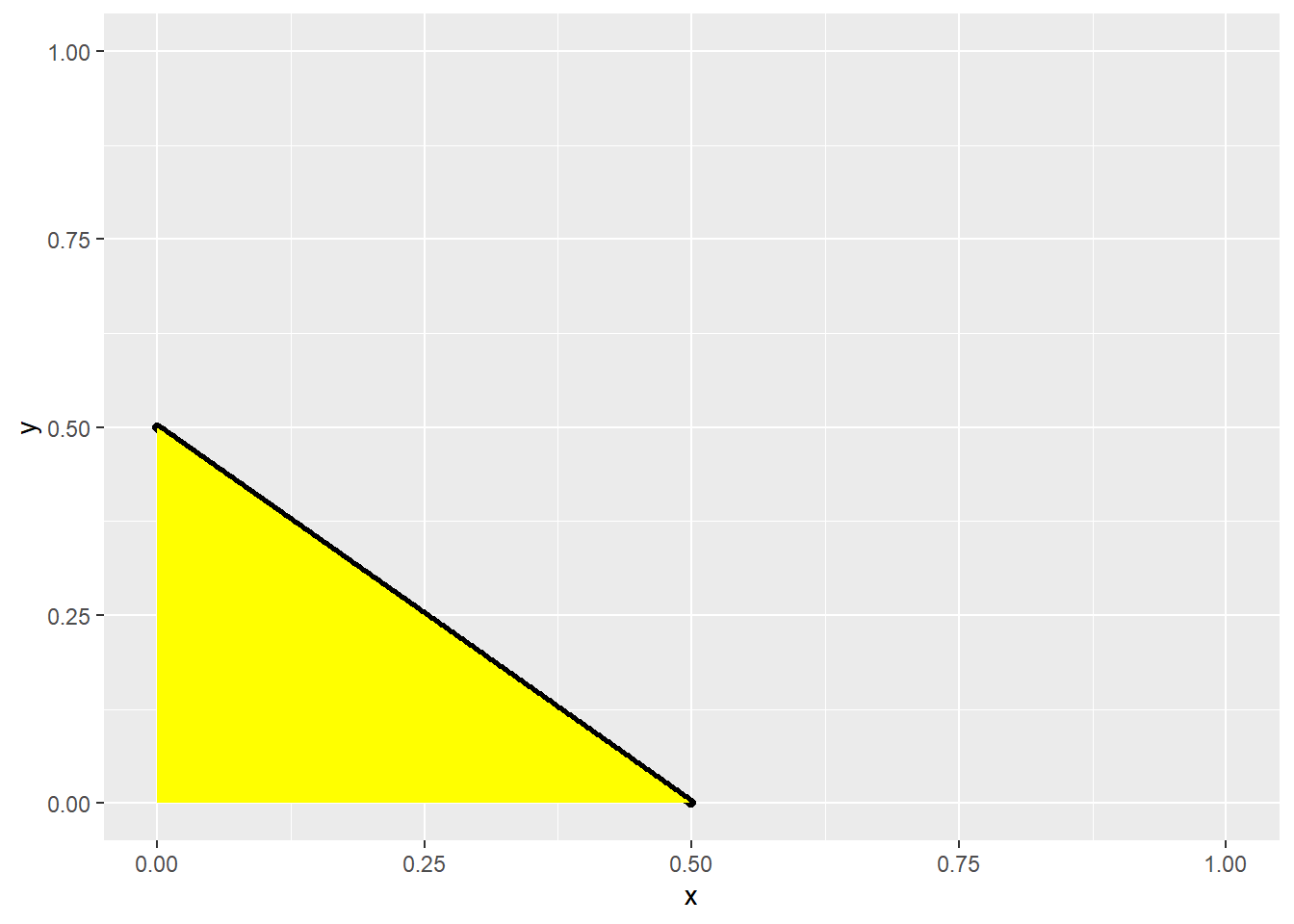The probability is given by $$\\p(a)\quad =\quad \int _{ 0 }^{ 1/2 }{ \left( -x\quad +\quad 1/2 \right) dx\quad =\quad 1/8 }\\$$

1. x*y < 1/2 means that y < 1/(2x) $$\\ p(b)\quad =\quad \int _{ 0 }^{ 1/2 }{ dx } +\quad \frac { 1 }{ 2 } \int _{ 1/2 }^{ 1 }{ \frac { 1 }{ x } dx } \quad =\quad \frac { 1 }{ 2 } \left( 1 +\left( { \left[ ln(x) \right] }_{ 1/2 }^{ 1 } \right) \right) \quad =\quad 1/2 +1/2ln2 = 0.846\\$$
df <- data.frame(x,y)
g <- ggplot(df, aes(x,y))
g + geom_area(fill = "Blue") + coord_cartesian(xlim = c(0,1), ylim = c(0,1))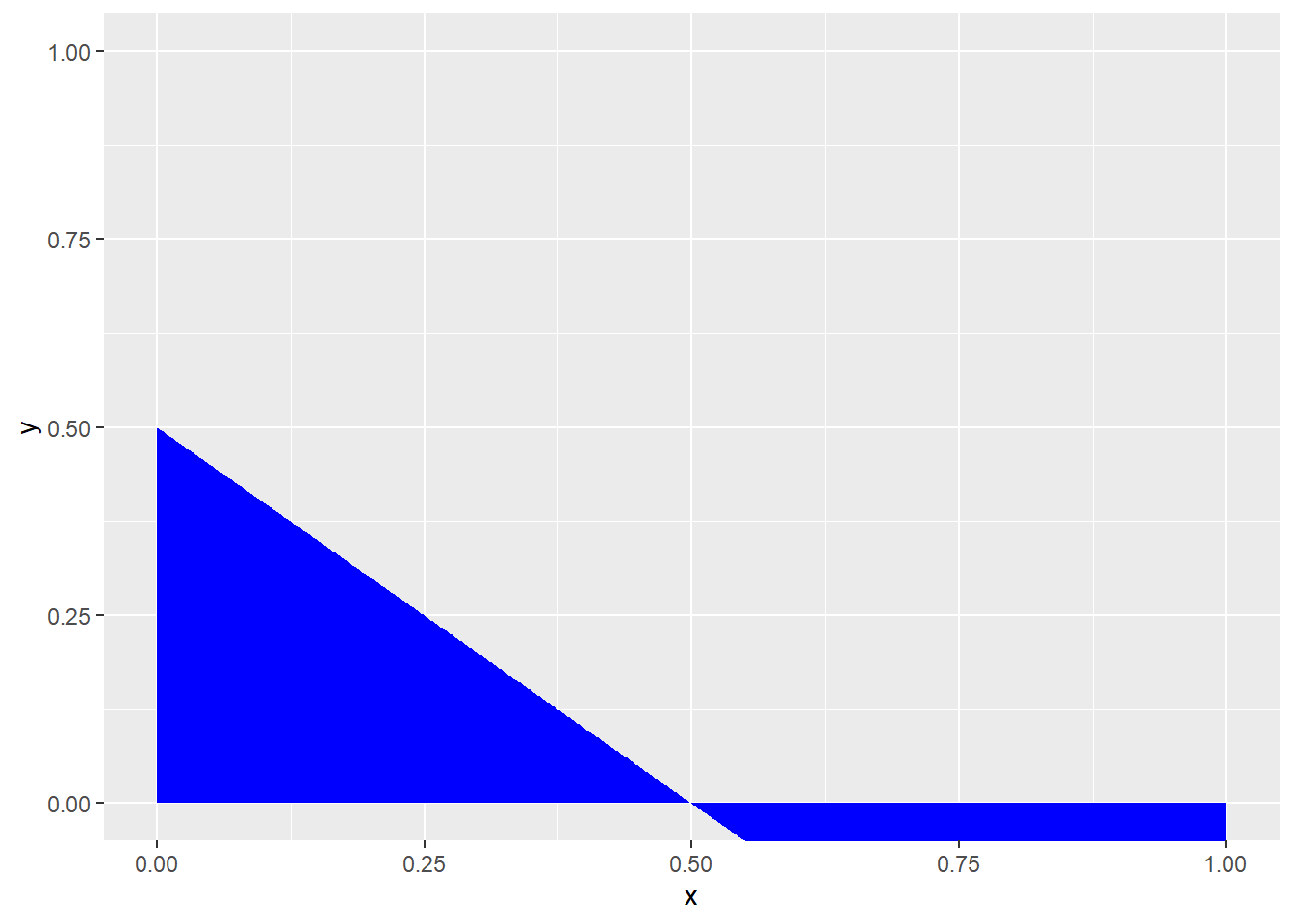y <- 1/(2*x)
qplot(x, y, xlim = c(0,1),ylim = c(0,1), color = I("Blue"))  + geom_area(fill = "Magenta")
## Warning: Removed 499 rows containing missing values (position_stack).
## Warning: Removed 499 rows containing missing values (geom_point).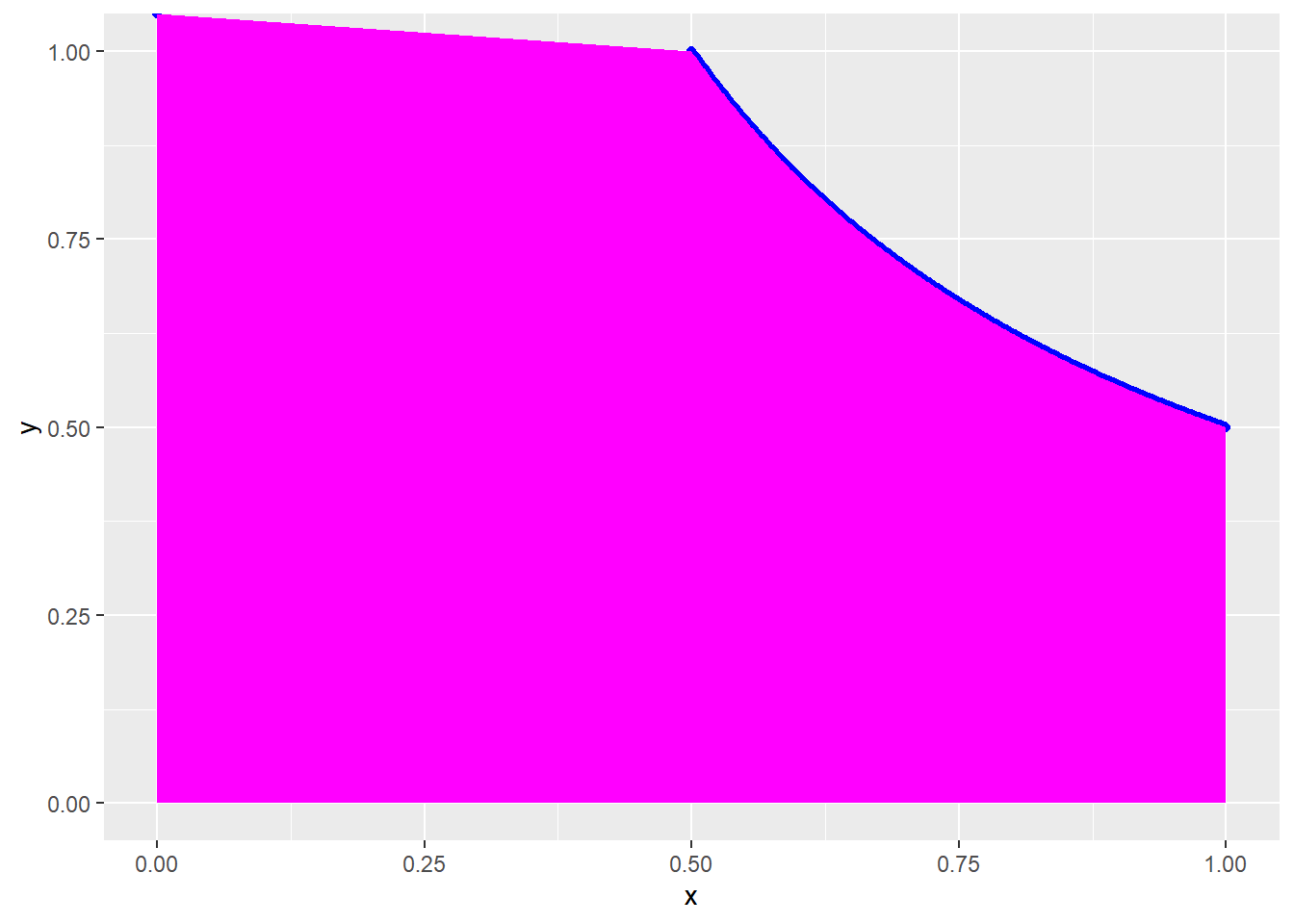1. |x-y| < 1/2 this implies =1/2 < y-x < 1/2 then x-1/2 < y < x + 1/2
y <- x + 1/2
y[501:length(x)] <- x[501:length(x)] - 1/2

qplot(x, y, xlim = c(0,1),ylim = c(0,1), color = I("Blue"))  + geom_area(fill = "Green")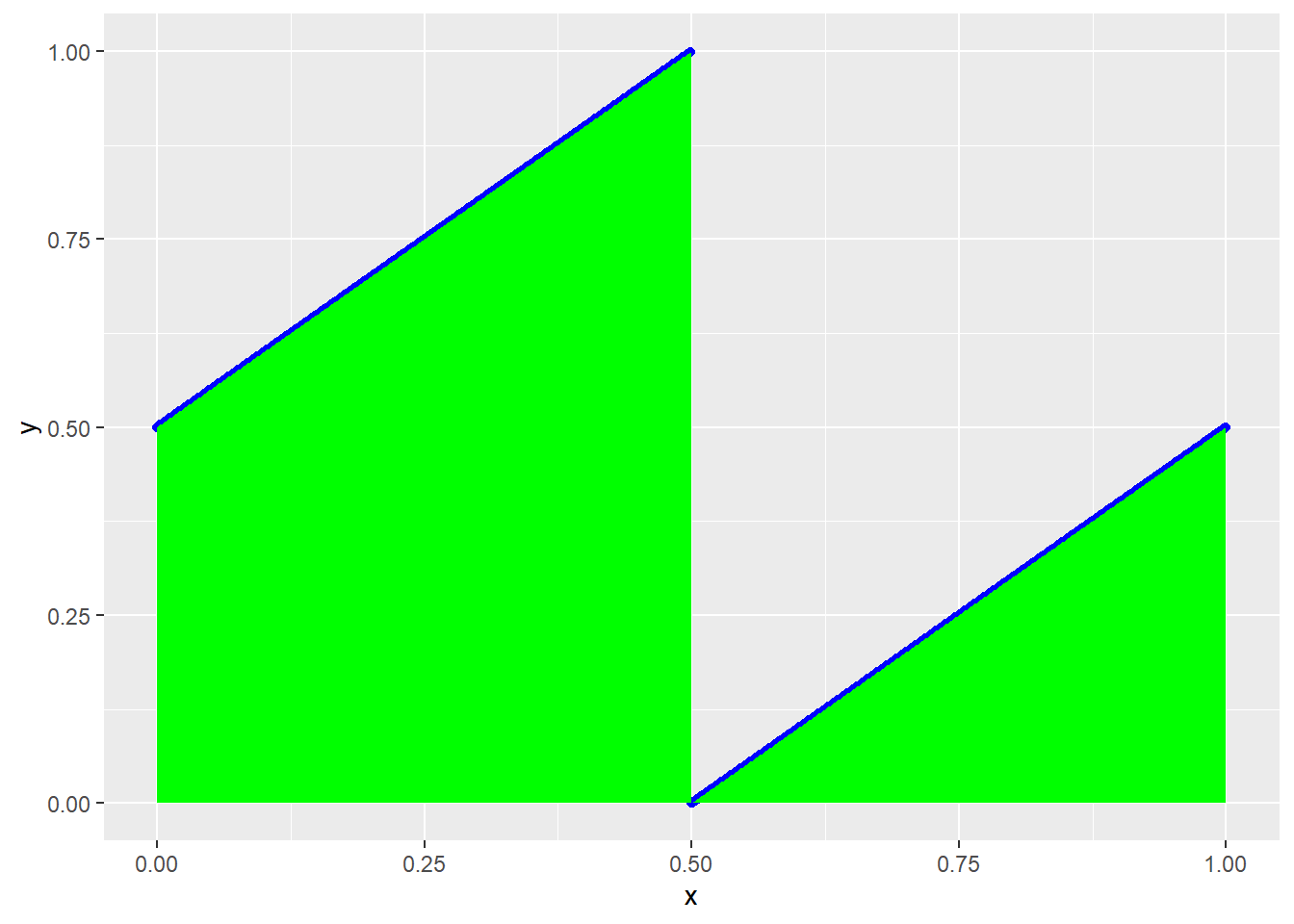The area between the upper blue-line and the x-axis and the area between the axis x = 1 and the lower blue=line represent our probability. ### p(c) = 1 = .5*.5 = .75

1. max(x,y) < 1/2
y[1:501] = 0.5
y[501:length(x)] <- 0
quickplot(x, y, xlim = c(0,1),ylim = c(0,1),color = I("Blue")) + geom_area(fill = "Blue")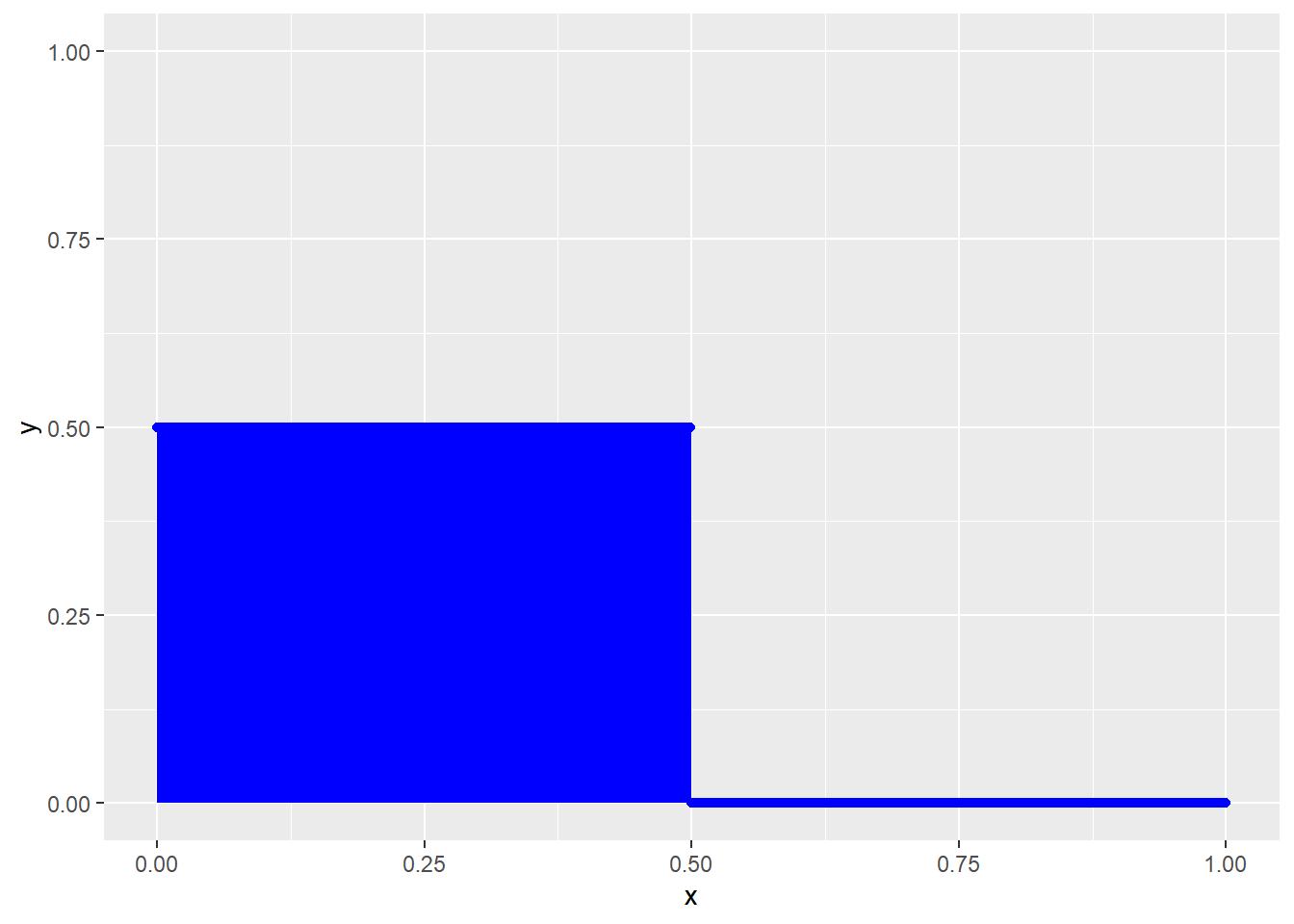The area between the horizontal lines and the x=axis represents our probability. ### p(d) = .5 *.5 = .25

1. min(x,y) < 1/2
y[1:501] = 1
y[501:length(x)] <- .5
quickplot(x, y, xlim = c(0,1),ylim = c(0,1),color = I("Blue")) + geom_area(fill = "Cyan")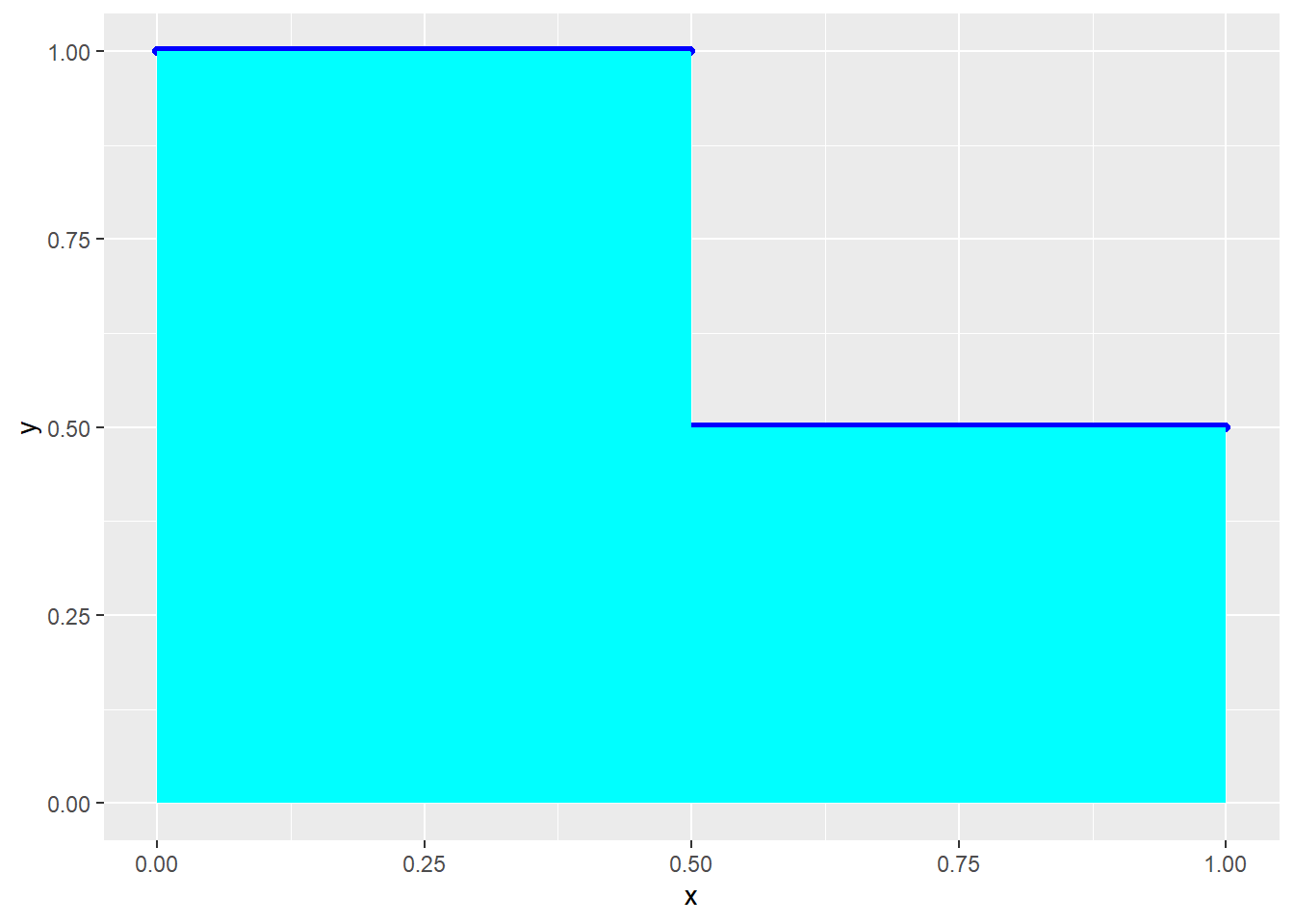The area between the horizontal lines and the x-axis is our probability. ### p(e) = .5x1 + .5x.5 = .75 or p(e) = 1 - .25 = .75